## Looking for your content...Type it here

### GATE Previous Years Papers on Instrumentation Engineering

GATE 2009 QUESTION PAPER IN: INSTRUMENTATION ENGG.

Q. 1 – Q20 carry one mark each.

1) if z=x+jy where x and y are real,the value of

(a) 1 (b)

2) The value of , where the contour of integration is a simple closed curve around the origin,is
(a)0
(b) 2πj
(c)
(d) 1/(2πj)

3) Let P ≠ 0 be a 3×3 real matrix. There exist linearly independent vectors x and y such that Px = 0 and Py = 0. The dimension of the range space of P is

(a)0
(b) 1
(c) 2
(d)3

4) A sphere of unit radius is centered at the origin. The unit normal at a point(x, y, z) on the surface of the sphere is the vector
(a) x,y,z
(b)
(c)
(d)

5) An LVDT is supplied with a sinusoidal voltage of amplitude 5V and frequency 1kHz. The output is connected to an ac voltmeter. The reading of the voltmeter is 1V for a displacement of 1mm from the null position. When the displacement is 1mm in the opposite direction from the null position, the reading of the voltmeter is
(A) 1V
(B) 0.2V
(C) 1V
(D)5V

6) The circuit shown is the figure is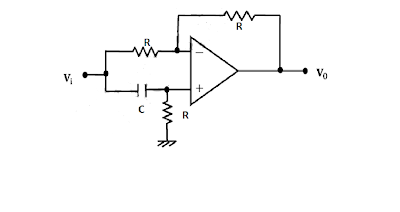(a) an all-pass filter
(b) a bandpass filter
(c) a highpass filter
(d) a lowpass filter

7) The diodes in the circuit shown are ideal. A voltage of 0V represents logic 0 and +5V represents logic 1. The logic function Z realized by the circuit for logic inputs X and Y is(a)Z = X = Y
(b) Z = XY
(c)

8) The minimal sum-of-products expression for the logic function f represented by the given Karnaugh map is9) In the figure shown, the initial state of Q is 0. The output is observed after the application of each clock pulse. The output sequence at Q is(a)0000…
(b) 1010…
(c) 1111…
(d)1000…

10) The binary representation of the decimal number 1.375 is
(a) 1.111
(b) 1.010
(c) 1.011
(d) 1.001

11) Consider a system consisting of a microprocessor, memory, and peripheral devices connected by a common bus. During DMA data transfer, the microprocessor
(a) only read from the bus
(b) only write to the bus
(c) both read from and writes to the bus
(d) neither read from nor writes to the bus

12) The fundamental period of x(t)=2sinπt + 3sin3πt, with t expressed in seconds, is
(A) 1s
(B) 0.67s
(C) 2s
(D) 3s

13) A linear time-invariant causal system has a frequency response given in polar form as . For input x(t) = sin t, the output is

14) A 50% duty cycle square wave with zero mean is used as a baseband signal in an ideal frequency modulator with a sinusoidal carrier of frequency . The modulated signal is given as an input to an ideal phase demodulator (a circuit that produces an output proportional to the difference in phase of the modulated signal from that of the carrier). The output of the circuit is
(A) a square wave
(B) a train of impulses with alternating signs
(C) a triangular wave
(D) a sinusoidal wave

15) The figure shows a periodic waveform to be displayed on a CRO. A trigger setting which ensures a stationary display is(A) level: 0.2V, slope:- ve
(B) level: 0.5V, slope:- ve
(C) level: 0.2V, slope: + ve
(D) level: 1.8V, slope:- ve

16) The input impedance of CRO is equivalent to a 1MΩ resistance in parallel with a 45pF capacitance. It is used with a compensated 10-to-1 attenuation probe. The effective input capacitance at the probe tip is
(A) 4.5 pF
(B) 5 pF
(C) 45 pF
(D)450 pF

17)A galvanometer with internal resistance 100Ω and full-scale current 1mA is used to realize a dc voltmeter with a full scale range of 1V. The full scale range of this voltmeter can be extended to 10V by connecting an external resistance of value
(A)9kΩ
(B) 9.9kΩ
(C) 10kΩ
(D)11kΩ

18) A plant with a transfer function is controlled by a PI controller with = 1 and ≥ 0 in a unity feedback configuration. The lowest value of that ensures zero steady state error for a step change in the reference input is
(A)0
(B) 1/3
(C) 1/2
(D)1

19) A mass spectrometer is to be used to resolve peaks corresponding to and The atomic masses are 12C12.0000, 16O = 15.9949, and 14
(A)250 N = 14.0031 amu. The resolving power of the mass spectrometer should be at least
(B) 350
(C) 2500
(D)3500

20) Assuming complete dissociation, the pH of a 1mM solution of is closest to
(A)0
(B) 2.7
(C) 2.4
(D) 2.1

21) The eigen values of a (2 × 2) matrix X are 2 and 3. The eigenvalues of matrix (X+5I) are
(A) 3,4
(B) 1,2
(C) 1,3
(D) 2,4

22) The matrix P = rotates a vector about the axis by an angle of
(A) 30º
(B) 60º
(C) 90º
(D) 120º

23) A screening test is carried out to detect a certain disease. It is found that 12% of the positive reports and 15% of the negative reports are incorrect. Assuming that the probability of a person getting a positive report is 0.01, the probability that a person tested gets an incorrect report is
(A) 0.0027
(B) 0.0173
(C) 0.1497
(D) 0.2100

24) One of the roots of the equation = j, where j is the positive square root of 1, is

25) The differential equation = , with x(0) = 0, and the constant 0 , is to be numerically integrated using the forward Euler method with a constant integration time step T. The maximum value of T such that the numerical solution of x converges is
(A)
(B)

26) A quantity x is calculated by using the formula,
The measured values are .Assume that the measurement errors in p, q and r are independent. The absolute maximum error in the measurement of each of the three quantities is . The absolute maximum error in the calculated value of x is

27) The response of a first order measurement system to a unit step input is , where t is in seconds. A ramp of 0.1units per second is given as the input to this system. The error in the measured value after transients have died down is
(A) 0.02units
(B) 0.1unitsThe source network S is connected to the load network L as shown by dashed lines.

28) The power transferred from S to L would be maximum when is(A)0Ω
(B) 0.6Ω
(C) 0.8Ω
(D)2Ω

29) A stroboscopic system is used for measuring the speed of a rotating shaft. The shaft has one target mark on it. The maximum strobe rate at which synchronism is achieved is flashes per minute. The next lower flash rate at which synchronism is achieved is flashes per minute. The speed of the shaft in rpm is

30) The figure shows the cross-sectional diagram of an orifice flow meter with an orifice radius R. Point ‘a’ is 30R upstream while points ‘b’ and ‘c’ are 0.8R and 30R downstream from the orifice respectively. The pressures at points a, b and c are , and respectively. Then(a)

31) The values of the material constant β for thermistors P and Q are 4000 K and 3000 K, respectively. The resistance of each thermistor at 298 K is 2kΩ. At 373 K the ratio of the resistance of thermistor P to that of thermistor Q will be closest to
(A) 1.33
(B) 1.00
(C) 0.75
(D) 0.50

32) Four strain gauges are fixed on a cylindrical shaft to measure torque, as shown in the figure.A correct way to place these gauges in the bridge is

33) In the circuit shown, the Zener diode has ideal characteristics and a breakdown voltage of 3.2V. The output voltage for an input voltage = +1V is closest to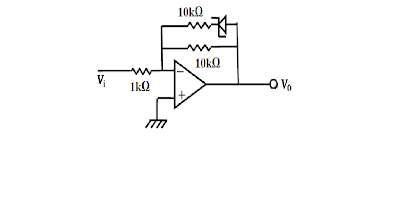(A) 10V
(B) 6.6V
(C) 5V
(D) 3.2V

34) The input resistance of the circuit shown in the figure, assuming an ideal op amp, is(A)R/3
(B) 2R/3
(C) R
(D)4R/3

35) In the circuit shown in the figure, the switch S has been in Position 1 for a long time. It is then moved to Position 2. Assume the Zener diodes to be ideal. The time delay between the switch moving to Position 2 and the transition in the output voltage is(A)5.00ms
(B) 8.75ms
(C) 10.00ms
(D)13.75ms

36)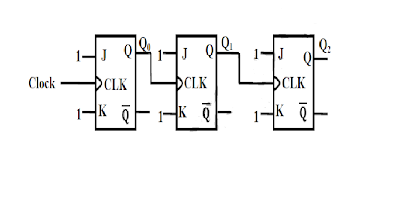The figure above shows a 3-bit ripple counter, with as the MSB. The flip-flops are rising-edge triggered. The counting direction is
(A) always down
(B) always up
(C) up or down depending on the initial state of only
(D) up or down depending on the initial states of and

37) 37. An 8-bit ADC, with 2’s complement output, has a nominal input range of 2V to + 2V. It generates a digital code of 00H for an analog input in the range 7.8125mV to +7.815mV. An input of 1.5V will produce a digital output of
(A)90H
(B) 96H
(C) 9BH
(D)A0H

38) The following is an assembly language program for 8085 microprocessors:

 Address Instruction Code Mnemonic 1000H 3E06 MVI A, 06H 1002H C6 70 ADI 70II 1004H 32 07 10 STA 1007H 1007H AF XRA 1008H 76 HLT

When this program halts, the accumulator contains
(A)00H
(B) 06H
(C) 70H
(D)76H

39) For input x(t), an ideal impulse sampling system produces the output
where is the Dirac delta function.
The system is
(A) nonlinear and time invariant
(B) nonlinear and time varying
(C) linear and time invariant
(D) linear and time varying

40) The root mean squared value of x(t) =3 + 2sin(t)cos(2t) is
(A)
(B)

41) An analog signal is sampled at 9kHz. The sequence so obtained is filtered by an FIR filter with transfer function . One of the analog frequencies for which the magnitude response of the filter is zero is
(A)0.75kHz
(B) 1kHz
(C) 1.5kHz
(D)2kHz

42) The transfer function H(z) of a fourth-order linear phase FIR system is given by

43) The dc potentiometer shown in the figure has a working current of 10mA with switch S open. Let + = 100Ω. The galvanometer G can only detect currents greater than 10 μ A. The maximum percentage error in the measurement of the unknown emf Ex as calculated from the slider position shown is closest to(A) 0.3
(B) 0.5
(C) 0.6
(D) 1.0

44) A filter is represented by the signal flow graph shown in the figure. Its input is x(t) and output is y(t). The transfer function of the filter is45) A unity feedback control loop with an open transfer function of the form has a gain crossover frequency of 1 rad/s and a phase margin of 60º. If an element having a transfer function is inserted into the loop, the phase margin will become
(A) 0º
(B) 30º
(C) 45º
(D) 60º

46) A linear time-invariant single-input single-output system has a state Pace model given by

Here x is the state vector, u is the input, and y is the output.
The damping ratio of the system is
(A) 0.25
(B) 0.5
(C) 1
(D)2

47) A unity feedback system has the transfer function
The value are for which the loci of all the three roots of the closed loop characteristic equation meet at a single point is
(A)10/9
(B) 20/9
(C) 30/9
(D)40/9

48) A standard three-lead frontal plane ECG is taken of a person with a normal heart. The peak amplitude of the R-wave is
(D) equal in all the leads

49) The operating voltage of an X-ray tube is changed from 40kV to 50kV. The resulting change in the shortest wavelength generated is
(A)+20%
(B) 20%
(C) +25%
(D) 36%

50) In a pulsed ultrasound imaging system, a single 5MHz crystal is used both as source and as detector. Bursts of at least 20 cycles are needed for acceptable image quality. The velocity of sound in the tissue being imaged is 1500m/s. The minimum distance of the objects to be imaged should be
(A)12mm
(B) 6mm
(C) 1mm
(D)3mm

Common Data Questions
Common Data for Questions 51 and 52:

The figure shows a sample-and-hold circuit using a MOSFET as a switch. The threshold voltage of the MOSFET is +2V. It has zero leakage current in the off state. Assume that the capacitor is ideal.51)The input voltage ranges from 5V to +5V. Appropriate values of , of during sampling, and of during hold are, respectively,
(A)12V, +7V and 3V
(B) 12V, +3V, and 7V
(C) +12V, +3V,and 7V
(D) 12V, +7V, and 3V

52) 52. The circuit is used at a sampling rate of 1kHz, with an A/D converter having a conversion time of 200μs. The op amp has an input bias current of 10mA. The maximum hold error is
(A)1mV
(B) 2mV
(C) 5mV
(D)10mV

Common Data for Questions 53 and 54:

The circuit shown in the figure uses three identical transistors with = 0.47V and β = 100. Given: =1kΩ, kT/ = 25mV. The collector current of transistor is 2mA.53)The bias voltage at the base of the transistor is approximately
(A) 9.3V
(B) 10.0V
(C) 10.3V
(D) 11.0V

54) The small signal voltage gain of the circuit is
(A) 20
(B) 40
(C) 20
(D)40

Common Data for Questions 55 and 56:

The figure shows an arrangement for measuring small angular displacements in a vertical plane. A non-conducting tube of length 2l and rectangular cross section (width w, height d) is bent along an arc of a circle with radius R >> d, centered at P. Four electrode plates of length l and width w are placed to form two curved parallel plate capacitors and with a negligible gap between them. The tube contains water with an air bubble of rectangular cross section (width w, height d) and length l/4. The capacitors are connected in a bridge circuit as shown in the figure, where the bridge has ac excitation . Angular displacement occurs about the point P.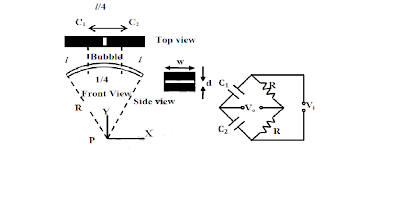55) The range of angular displacement (in radians) this system can measure is

56) The sensitivity is
(A) inversely proportional to R and l
(B) inversely proportional to R and directly proportional to l
(C) directly proportional to R and l
(D) directly proportional to R and inversely proportional to l

Common Data for Questions 57 to 58:

A disturbance input d(t) is injected into the unity feedback control loop shown in the figure. Take the reference input r(t) to be a unit step.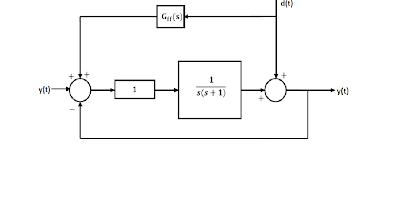57) If the disturbance is measurable, its effect on the output can be minimized significantly using a feedforward controller ). To eliminate the component of the output due to d(t) = sin t, should be

58) Let (s) be a PD controller. If d(t) = sin 2t, the amplitude of the frequency component of y(t) due to d(t) is

A Michelson interferometer illuminated with a source of central wavelength and spectral width of is adjusted for equal path difference for the beams returning from the two mirrors. When one of the mirrors is moved by a distance of 0.1mm from this position, 300 fringes move past the field of view. When the mirror is moved further, the fringes completely disappear when the mirror is approximately 4cm from the initial position.

59) The central wavelength of the source is
(A)540nm
(B) 632.8nm
(C) 667nm
(D)720nm

60)The spectral width of the source is approximately
(A)0.0056nm
(B) 0.0100nm
(C) 0.0500nm
(D)0.1000nm